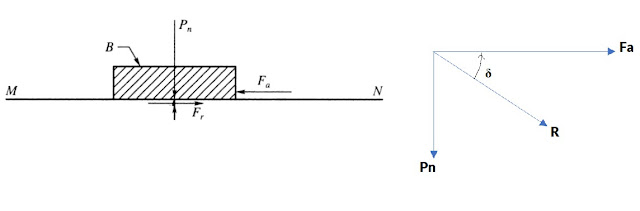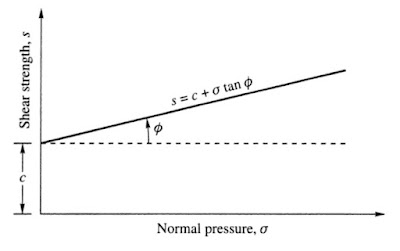# Shear Strength of Soil

The shear strength of soil can be defined as its maximum resistance to shear stress just before the failure.

Shear strength is one of the three principal engineering properties of soil (permeability, compressibility, and shear strength) which controls the stability of soils for different kinds of slope formation under different load actions. In contrast, if considering water; water does not possess shear strength and hence cannot form a slope.

Almost all problems related to soil engineering are related to the shear strength of the soil. It is the primary factor studied to understand soil-structure interactions and for stability analysis during the design of foundations, retaining walls, stability of earth slopes, etc.

This article series is exclusively created to explain the basic concept of the shear strength of soils along with their characteristics and theories employed in soil engineering.

### Shear Strength of Soil: Basics of Soil Mechanics

The soil shear strength is a measure of soil resistance against shear stresses. Shear stresses in the soil are caused due to direct tension or direct compression.

Soil subjected to direct tension results in shear stresses which would result in tension failure in the form of cracks and fissures. While the soil under direct compression results in shear stresses that might result in shear failure.

Hence, direct tension shear stress is not relevant compared to shear stresses due to direct compression. So we can say, the shear failure of a soil mass occurs when the shear stresses induced due to the applied compressive loads exceed the shear strength of the soil.

When we say the shear failure of soil, it implies the failure of the soil due to the relative movements of the particles and not the breaking of the particles.

### Factors Affecting Shear Strength of Soil

We now understood that shear strength is a complex parameter of soil which is in turn a complex function of several contributing factors, that includes:

1. Friction resistance between particles [Sliding Friction & Rolling Friction]
2. The interlocking of the particles
3. Interparticle cohesion

These three parameters differ from soil to soil. The shear strength of clayey soil is not similar to that of sandy soil. Granular soil possesses shear strength due to its frictional resistance and particle interlocking. While in the case of cohesive soils, shear strength is due to cohesion between the soil particle.

### Shearing Resistance & Shearing Strength of Soil

The behavior of soil under the action of stress is studied to develop a relation to easily understand the failure mechanism of soil structure under varied load action. To study this, we consider the soil mass block B subjected to a stress system.

Note: Stress systems in most studies are considered to be three-dimensional. As for soil engineering problems, the stress acting in the third direction is not relevant, the stress system is considered to be two-dimensional.Figure-1

Consider a block B subjected to a normal force Pn acting normally on the surface MN and a force Fa acting in a tangential direction as shown in Figure 2.

The normal force Pn remains constant while the value of Fa is increasing from a value of zero to a value until it slides block B.

The action of Fa force results in an equal and opposite force Fr, which is caused due to the friction between the contact surface between the block and MN. The force Fr balances the Fa and keeps the block steady.

The forces Pn and Fr result in a resultant force R that makes an angle δ with the normal to the plane MN, as explained in the force diagram (Figure 2 (b)). The angle δ is called the angle of obliquity.

Block B starts to slide when Fa increases, and the value of δ reaches a maximum value of δm.

In the experiment conducted, block B and surface MN may or may not be made out of the same material. If they are made of the same material, then δm is equal to the angle of friction (φ). Then tan φ is the angle of friction.

Then, the applied force Fa is the shearing force and the force of friction Fr is the shearing resistance. If so,

The maximum shearing resistance that the materials are capable of developing is called shear strength.

### Shear Strength of Soil Equation

The above experiment can be performed for a higher value of normal force (Pn) which will result in a higher value of Fa. Hence, from numerous experiments, it is studied that, Fa is directly proportional to the normal force Pn.

Fa α Pn

Fa = Pn tan φ ( EQ.1)

If the contact area of block B is ‘A’, then,

shear strength s = force/area = Fa/A = [ Pn/A ] tan φ   [EQ.2]   [ from EQ.1]

normal stress σ =  normal force/ area = Pn /A

After substituting in EQ.2

s = σ tan φ [EQ.3]

### Coulomb Shear Strength Equation

The fundamental shear strength property of soil was first recognized by Coulomb and he expressed the shear strength of soil as a linear function of normal stress:

s= c + σ. tan φ                              [EQ.4]

The shear strength equation s = σ tan φ, is applied for soil with friction alone i.e. granular soils. All soils are not purely granular. They will have an additional strength contributed by the property of cohesion between soil particles.

Hence, the strength of soil is contributed by two components. One is due to the cohesion between the soil particles (c) and the other one is due to the friction between them (φ).

In the above equation, S is the shear strength, c is the cohesion, φ is the angle of shearing resistance and σ is the normal stress. The equation expresses the assumption that cohesion c is independent of the normal pressure σ acting on the plane of failure.Figure-2: Coulomb's Law

Coulomb’s law and the relationship between the various parameters can be represented by a graph in Figure 2.

• When the value of normal force (Pn ) is zero, the normal pressure σ = 0; then s = c. [ It makes a y-intercept at the y-axis]. This defines, the cohesion of soil is defined as the shearing strength at zero normal pressure on the plane of rupture.
• With the increase in the value of normal pressure, the shear strength increase line is shown in the graph.

### Features of Coulomb’s Equation

The c and φ are empirical parameters present in Coulomb’s equation.

These empirical parameters are dependent on the history of the soil, the initial condition of the soil (saturated or unsaturated), the permeability characteristics of the soil, and the drainage conditions allowed in the soil.

For cohesion less soil c=0; then Coulomb’s equation becomes s = σ tan φ.

The shear strength of the soil is determined by laboratory methods and field methods. These test methods determine the shear strength parameters c and φ of the soils and thereby calculate the shear strength of the soil at the site.